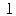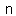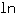# U+33D1 SQUARE LN

U+33D1 was added to Unicode in version 1.1 (1993). It belongs to the blockCJK Compatibility in the Basic Multilingual Plane.

This character is a Other Symbol and is commonly used, that is, in no specific script.

The Wikipedia has the following information about this codepoint:

The natural logarithm of a number is its logarithm to the base e, where e is an irrational and transcendental constant approximately equal to 7000271828182845899♠2.718281828459. The natural logarithm of x is generally written as ln x, loge x, or sometimes, if the base e is implicit, simply log x. Parentheses are sometimes added for clarity, giving ln(x), loge(x) or log(x). This is done in particular when the argument to the logarithm is not a single symbol, to prevent ambiguity.

The natural logarithm of x is the power to which e would have to be raised to equal x. For example, ln(7.5) is 2.0149..., because e2.0149... = 7.5. The natural log of e itself, ln(e), is 1, because e1 = e, while the natural logarithm of 1, ln(1), is 0, since e0 = 1.

The natural logarithm can be defined for any positive real number a as the area under the curve y = 1/x from 1 to a (the area being taken as negative when a<1). The simplicity of this definition, which is matched in many other formulas involving the natural logarithm, leads to the term "natural". The definition of the natural logarithm can be extended to give logarithm values for negative numbers and for all non-zero complex numbers, although this leads to a multi-valued function: see Complex logarithm.

The natural logarithm function, if considered as a real-valued function of a real variable, is the inverse function of the exponential function, leading to the identities:

Like all logarithms, the natural logarithm maps multiplication into addition:

Thus, the logarithm function is an isomorphism from the group of positive real numbers under multiplication to the group of real numbers under addition, represented as a function:

Logarithms can be defined to any positive base other than 1, not only e. However, logarithms in other bases differ only by a constant multiplier from the natural logarithm, and are usually defined in terms of the latter. For instance, the binary logarithm is the natural logarithm divided by ln(2), the natural logarithm of 2. Logarithms are useful for solving equations in which the unknown appears as the exponent of some other quantity. For example, logarithms are used to solve for the half-life, decay constant, or unknown time in exponential decay problems. They are important in many branches of mathematics and the sciences and are used in finance to solve problems involving compound interest.

## Representations

System Representation
13265
UTF-8 E3 8F 91
UTF-16 33 D1
UTF-32 00 00 33 D1
URL-Quoted %E3%8F%91
HTML-Escape &#x33D1;
Wrong windows-1252 Mojibake ã

## Complete Record

Property Value
Age (age) 1.1
Unicode Name (na) SQUARE LN
Unicode 1 Name (na1) SQUARED LN
Block (blk) CJK_Compat
General Category (gc) Other Symbol
Script (sc) Common
Bidirectional Category (bc) Left To Right
Combining Class (ccc) Not Reordered
Decomposition Type (dt) Square
Decomposition Mapping (dm)Lowercase (Lower)
Simple Lowercase Mapping (slc)Lowercase Mapping (lc)Uppercase (Upper)
Simple Uppercase Mapping (suc)Uppercase Mapping (uc)Simple Titlecase Mapping (stc)Titlecase Mapping (tc)Case Folding (cf)ASCII Hex Digit (AHex)
Alphabetic (Alpha)
Bidi Control (Bidi_C)
Bidi Mirrored (Bidi_M)
Bidi Paired Bracket (bpb)Bidi Paired Bracket Type (bpt) None
Cased (Cased)
Composition Exclusion (CE)
Case Ignorable (CI)
Full Composition Exclusion (Comp_Ex)
Changes When Casefolded (CWCF)
Changes When Casemapped (CWCM)
Changes When NFKC Casefolded (CWKCF)
Changes When Lowercased (CWL)
Changes When Titlecased (CWT)
Changes When Uppercased (CWU)
Dash (Dash)
Deprecated (Dep)
Default Ignorable Code Point (DI)
Diacritic (Dia)
East Asian Width (ea) Wide
Extender (Ext)
FC NFKC Closure (FC_NFKC)Grapheme Cluster Break (GCB) Any
Grapheme Base (Gr_Base)
Grapheme Extend (Gr_Ext)
Hex Digit (Hex)
Hangul Syllable Type (hst) Not Applicable
Hyphen (Hyphen)
ID Continue (IDC)
Ideographic (Ideo)
ID Start (IDS)
IDS Binary Operator (IDSB)
IDS Trinary Operator and (IDST)
InMC (InMC)
Indic Positional Category (InPC) NA
Indic Syllabic Category (InSC) Other
ISO 10646 Comment (isc)
Joining Group (jg) No_Joining_Group
Join Control (Join_C)
Jamo Short Name (JSN)
Joining Type (jt) Non Joining
Line Break (lb) Ideographic
Logical Order Exception (LOE)
Math (Math)
Noncharacter Code Point (NChar)
NFC Quick Check (NFC_QC) Yes
NFD Quick Check (NFD_QC) Yes
NFKC Casefold (NFKC_CF)NFKC Quick Check (NFKC_QC) No
NFKD Quick Check (NFKD_QC) No
Numeric Type (nt) None
Numeric Value (nv) NaN
Other Alphabetic (OAlpha)
Other Default Ignorable Code Point (ODI)
Other Grapheme Extend (OGr_Ext)
Other ID Continue (OIDC)
Other ID Start (OIDS)
Other Lowercase (OLower)
Other Math (OMath)
Other Uppercase (OUpper)
Pattern Syntax (Pat_Syn)
Pattern White Space (Pat_WS)
Quotation Mark (QMark)
Radical (Radical)
Sentence Break (SB) Other
Simple Case Folding (scf)Script Extension (scx) Common
Soft Dotted (SD)
STerm (STerm)
Terminal Punctuation (Term)
Unified Ideograph (UIdeo)
Variation Selector (VS)
Word Break (WB) Other
White Space (WSpace)
XID Continue (XIDC)
XID Start (XIDS)
Expands On NFC (XO_NFC)
Expands On NFD (XO_NFD)
Expands On NFKC (XO_NFKC)
Expands On NFKD (XO_NFKD)